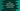# C++ program to find the largest of four numbers using class## C++ program to find the largest of four numbers using class:

In this post, we will learn how to find the largest of four numbers using a class and with a function in the class. The same code can be used to solve this problem by using a separate function without any class.

With this program, you will learn how to find the largest of four different numbers and how to use classes in C++.

### C++ program:

Classes can be used to group similar contents. For example, we can create one utility class and put different utility methods in that class.

For a large project, we can use that same utility class from different parts of the project. It makes the codebase concise and organized.

Below is the complete C++ program that uses a class to find the largest of four numbers:

``````#include <iostream>
using namespace std;

class MaxCalculator
{
private:
int first, second, third, fourth, max;

public:
void findMax();
void showMax();
};

void MaxCalculator::findMax()
{
cout << "Enter the first number : " << endl;
cin >> first;

cout << "Enter the second number : " << endl;
cin >> second;

cout << "Enter the third number : " << endl;
cin >> third;

cout << "Enter the fourth number : " << endl;
cin >> fourth;

int maxFirst = first > second ? first : second;
int maxSecond = third > fourth ? third : fourth;

max = maxFirst > maxSecond ? maxFirst : maxSecond;
}

void MaxCalculator::showMax()
{
cout << "Maximum value : " << max << endl;
}

int main()
{
MaxCalculator calculator;
calculator.findMax();
calculator.showMax();
}``````

#### Explanation:

In this program,

• MaxCalculator class is used to find the maximum of four numbers.
• This class has five private int variables. first, second, third and fourth to hold the four user input numbers and max to hold the largest of these numbers. These are private, so we can’t access them from a different class.
• Two public functions are defined : findMax to take user inputs for the numbers and to find the largest value and showMax to print the largest value. As these are public, we can access them from a different class.
• Inside findMax, it is reading the user inputs for the numbers and calculates the maximum of these numbers. maxFirst finds the largest of first and second. maxSecond finds the largest of third and fourth. The largest of maxFirst and maxSecond is the largest of all of these four numbers.
• showMax is used to just print the maximum or largest value i.e. max.

### Sample output:

This program will print outputs like below:

``````Enter the first number :
1
Enter the second number :
2
Enter the third number :
3
Enter the fourth number :
4
Maximum value : 4``````